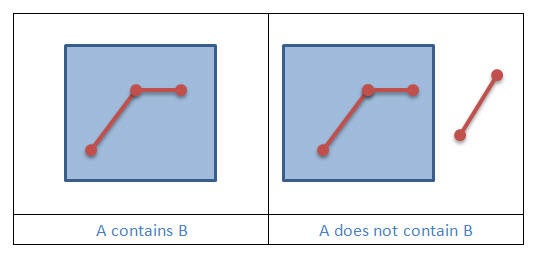One geometry contains another if the other geometry is a subset of it and their interiors have at least one point in common. Contains is the inverse of Within.

In the following pictures Geometry A is blue, and Geometry B is red.#### Example 1: Does Geometry A contain Geometry B?

```static boolean geometryContains(Geometry geometryA, Geometry geometryB, SpatialReference sr)
{
boolean contains = OperatorContains.local().execute(geometryA, geometryB, sr, null);
return contains;
}
```